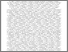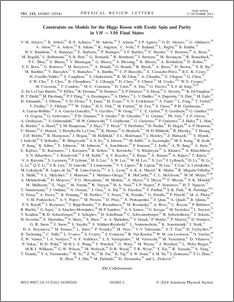# Constraints on models for the Higgs Boson with exotic spin and parity in \$VH\$Vbb\$ final states

, D0 Collaboration (2014) Constraints on models for the Higgs Boson with exotic spin and parity in \$VH\$Vbb\$ final states. Physical review letters, 113. ISSN 1079-7114Preview
PDF (PhysRevLett.113.161802)
PhysRevLett.113.161802.pdf - Published Version

## Abstract

We present constraints on models containing non-standard-model values for the spin J and parity P of the Higgs boson H in up to 9.7  fb−1 of pp¯ collisions at s√=1.96  TeV collected with the D0 detector at the Fermilab Tevatron Collider. These are the first studies of Higgs boson JP with fermions in the final state. In the ZH→ℓℓbb¯, WH→ℓνbb¯, and ZH→ννbb¯ final states, we compare the standard model (SM) Higgs boson prediction, JP=0+, with two alternative hypotheses, JP=0− and JP=2+. We use a likelihood ratio to quantify the degree to which our data are incompatible with non-SM JP predictions for a range of possible production rates. Assuming that the production rate in the signal models considered is equal to the SM prediction, we reject the JP=0− and JP=2+ hypotheses at the 97.6% CL and at the 99.0% CL, respectively. The expected exclusion sensitivity for a JP=0− (JP=2+) state is at the 99.86% (99.94%) CL. Under the hypothesis that our data are the result of a combination of the SM-like Higgs boson and either a JP=0− or a JP=2+ signal, we exclude a JP=0− fraction above 0.80 and a JP=2+ fraction above 0.67 at the 95% CL. The expected exclusion covers JP=0− (JP=2+) fractions above 0.54 (0.47).

Item Type:
Journal Article
Journal or Publication Title:
Physical review letters
Uncontrolled Keywords:
/dk/atira/pure/subjectarea/asjc/3100
Subjects:
Departments:
ID Code:
71417
Deposited By:
Deposited On:
24 Oct 2014 12:36
Refereed?:
Yes
Published?:
Published